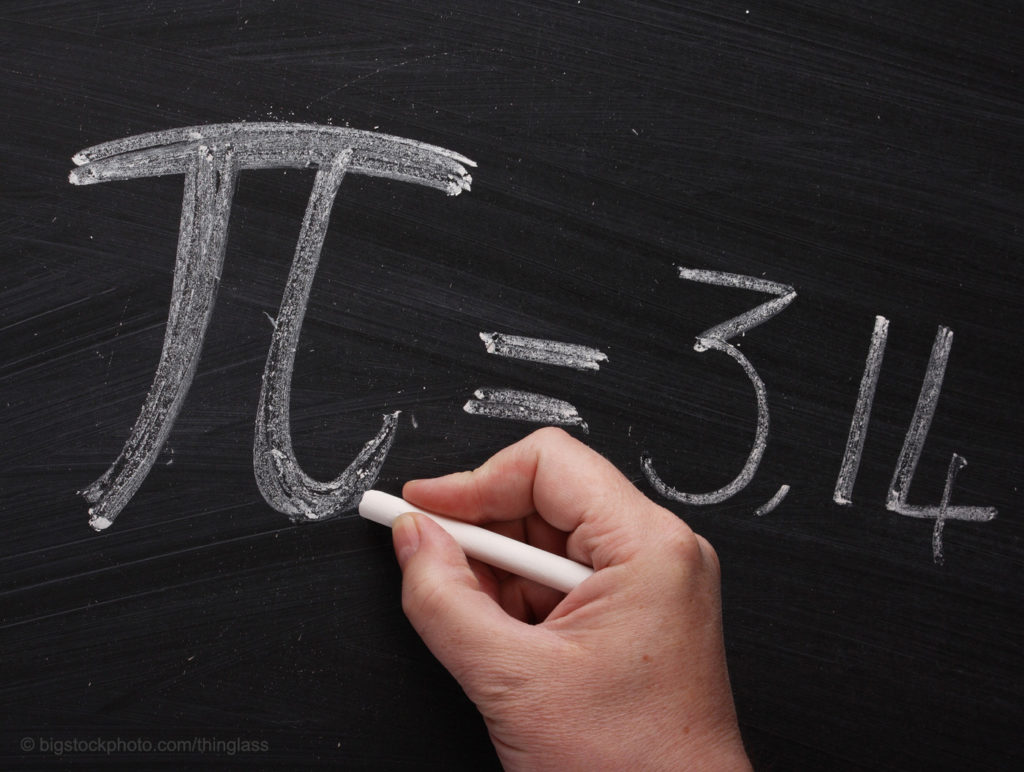# Cubit Pi SillinessThere is no limit to the extremes that skeptics will go trying to find mistakes in the Bible. One recent case involves cubit pi. An atheist claimed that the Bible was full of mathematical mistakes and impossibilities indicating the primitive nature and lack of knowledge of the authors. The case used was 1 Kings 7:23-26 and 2 Chronicles 4:2,5 where a laver is described, and numbers are given for its dimensions in cubits. The Bible says that the circumference of the round laver was 30 cubits and that the diameter was 10 cubits from rim to rim. The formula for the circumference of a circle is pi (3.14) times the diameter, so in this case it would be 31.4 cubits, not 30. A Hebrew cubit was 17.5 inches, a Babylonian cubit was 19.8 inches, and an Egyptian cubit was 20.63 inches, so this seems to be about a 19-inch mistake.

One might suggest that the rounding of numbers is the issue here since ancient measuring devices didn’t measure to two decimal places. But there is another interesting possibility. The ten cubits is measured rim to rim which would mean that the outside circumference was 31.4 cubits. However, if 30 cubits is the inside circumference of the laver, that would make the inside diameter 9.55 cubits. Subtracting 9.55 cubits from 10 would leave a difference of .45 cubits. So the thickness of the walls of the laver would be one-half of .45 cubits or .225 cubits (about 3.8 inches). First Kings 7:26 and 2 Chronicles 4:5 states that the walls of the laver were a “handbreadth” in thickness. Excavations of artifacts from ancient times frequently find lavers with that thickness, so the numbers are totally reasonable.

This is just another example of how desperate skeptics are to discard God and His Word. First Peter 3:15 tells us to be ready to give an answer to anyone who asks the reason for our faith, but some questions seem too silly to dignify by attempting an answer. This cubit pi challenge may be one of those questions, but we have an answer anyway.